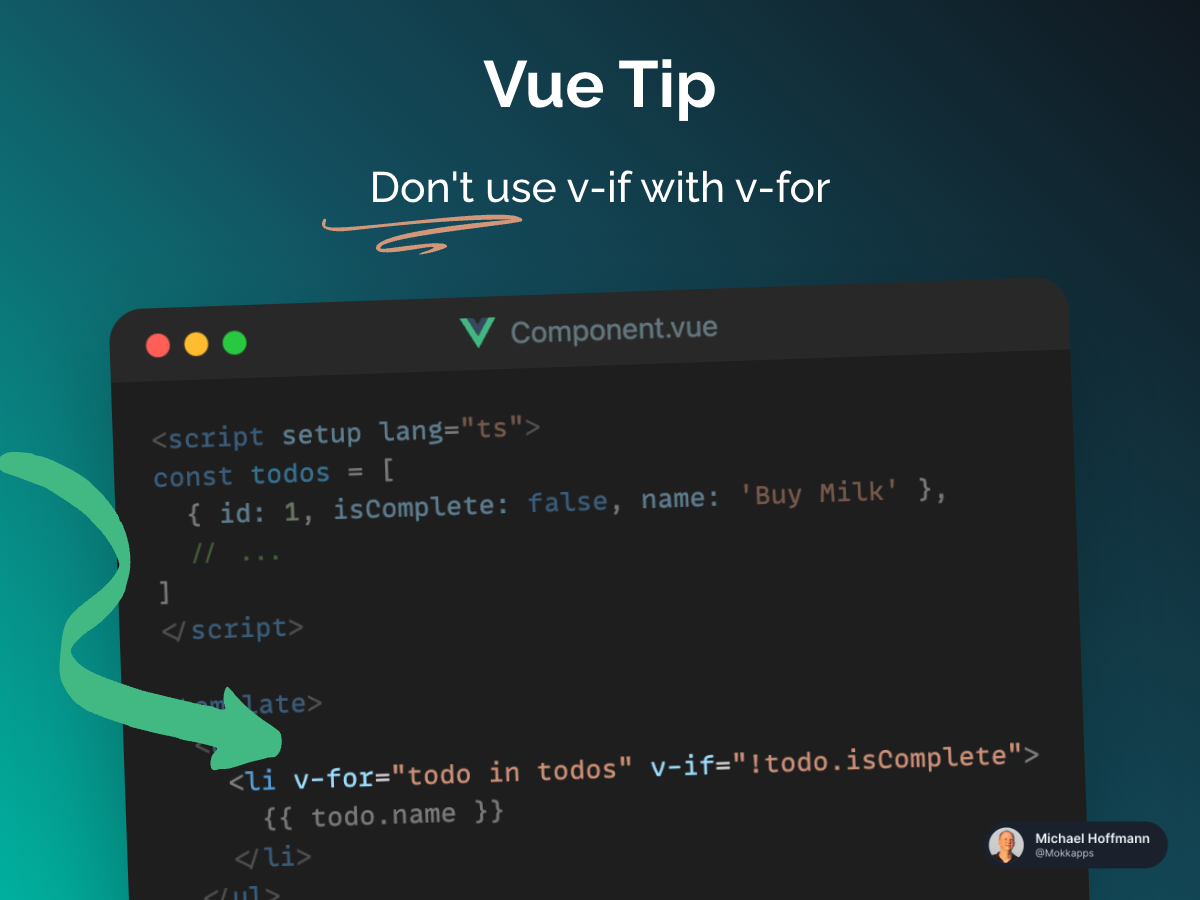Javascript is requiredMichael Hoffmann

# Vue Tip: Don't Use v-if With v-forMichael Hoffmann
Jun 16, 2023
|
163 views
TemplateIt can be tempting to use `v-if` with `v-for` in these common scenarios: Filtering items in a list and avoiding rendering a list if it should be hidden.

Let's take a detailed look at these two scenarios.

## Filtering items in a list

You might want to write the following code to filter items in a list:

Component.vue
``````
1
<script setup lang="ts">

2
const todos = [

3
{ id: 1, isComplete: false, name: 'Buy Milk' },

4
// ...

5
]

6
</script>

7

8
<template>

9
<ul>

10
<li v-for="todo in todos" v-if="!todo.isComplete">

11
{{ todo.name }}

12
</li>

13
</ul>

14
</template>
``````

This code will throw an error because the `v-if` directive will be evaluated first and the iteration variable `todo` does not exist at this moment. When Vue processes directives, `v-if` has a higher priority than `v-for`.

You can fix the code by using a computed property that returns your filtered list:

Component.vue
``````
1
<script setup lang="ts">

2
import { computed } from 'vue'

3

4
const todos = [

5
{ id: 1, isComplete: false, name: 'Buy Milk' },

6
// ...

7
]

8
const uncompletedTodos = computed(() => todos.filter((todo) => !todo.isComplete))

9
</script>

10

11
<template>

12
<ul>

13
<li v-for="todo in uncompletedTodos" :key="todo.id">

14
{{ todo.name }}

15
</li>

16
</ul>

17
</template>
``````

In this example, the code doesn't loop through the entire array, only the filtered computed property, which is also cached. In case you have massive arrays, this can be a huge performance improvement.

## Avoid rendering a list if it should be hidden

In some cases, you want to render a list only if a certain variable is set:

Component.vue
``````
1
<script setup lang="ts">

2
import { ref } from 'vue'

3

4
const todos = [

5
{ id: 1, isComplete: false, name: 'Buy Milk' },

6
// ...

7
]

8
const showTodos = ref(false)

9
</script>

10

11
<template>

12
<ul>

13
<li v-for="todo in todos" v-if="!showTodos">

14
{{ todo.name }}

15
</li>

16
</ul>

17
</template>
``````

A better solution would be to move the `v-if` to a container element (e.g. `ul`, `ol`):

Component.vue
``````
1
<script setup lang="ts">

2
import { ref } from 'vue'

3

4
const todos = [

5
{ id: 1, isComplete: false, name: 'Buy Milk' },

6
// ...

7
]

8
const showTodos = ref(false)

9
</script>

10

11
<template>

12
<ul v-if="!showTodos">

13
<li v-for="todo in todos">

14
{{ todo.name }}

15
</li>

16
</ul>

17
</template>
``````